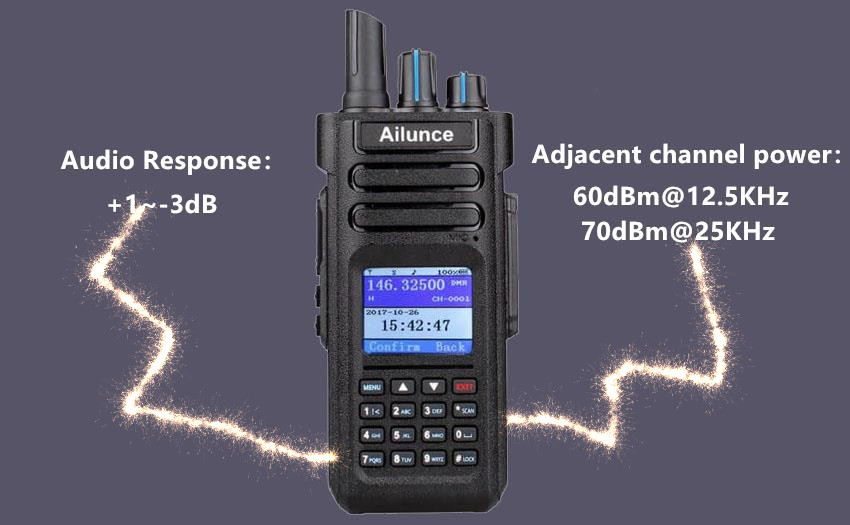# What's the meaning of dB in amateur radio?When talking about the gain of antennas and power amplifiers, the dB is often mentioned, but do you know what it means and how do we use it?

We often use the dB when measuring the volume. That's because it can be used as a unit of sound pressure level, which is approximately equal to the smallest division value at which the human ear usually perceives the difference in loudness. But this is actually just one use of dB. In fact, in addition to measuring sound intensity, dB is another way of expressing multiples.

When the dB is used as a multiple, there is no unit. When expressing the ratio of power, it is equal to 10 times the common logarithm of the ratio of power intensity. For example, N times the power conversion, dB is: N=10㏒10 (PB/PA), that is, the power of 10 is N, and the converted dB is expressed as (10*N) dB; when the ratio of the field quantity is expressed, it is equal to the field The ratio of strong amplitude is 20 times the common logarithm.
Let's take the real amateur radio exam as an example to practice the dB usage.

The ratio of output power and input power is 1/100, and the gain of the circuit is: -20dB

The ratio of output voltage and input voltage is 1/100, and the gain of the circuit is:  -40dB

Do you know what's meaning of dB in different scenarios?